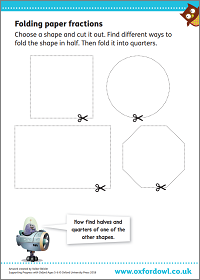We use cookies to enhance your experience on our website. By continuing to use our website, you are agreeing to our use of cookies. You can change your cookie settings at any time. Find out more
0 Items
Select Page

# Fractions, decimals & percentages

Understanding what fractions, decimals, and percentages mean is essential to be able to calculate and solve maths problems quickly. They will also come up all the time in later life, from dealing with money to cooking.

At the end of primary school, children sit mandatory tests in arithmetic and reasoning. Although these may seem a bit daunting, children build up to these by slowly improving their maths skills through the primary years. So, your child will progress from simple tasks like recognising halves and quarters in Year 1 to multiplying and dividing with fractions and decimals in Year 6.

There are a variety of simple things you can do at home to support your child’s developing understanding of fractions, decimals, and percentages.

## What your child will learn

Follow the links below for an overview of each year, with lots of information, support, and practice activities:

#### Fractions in Year 1 (age 5–6)

In Year 1, your child will recognise halves and quarters of objects, shapes, and quantities. This includes:

• understanding halving as sharing equally into two parts or groups
• understanding finding a quarter as sharing into four equal parts or groups
• knowing that finding a half is the same as dividing by 2 and finding a quarter is the same as dividing by 4.

#### Fractions in Year 2 (age 6–7)

In Year 2, your child will be expected to recognise and use the fractions , , and . This includes:

• finding fractions of lengths, shapes, sets of objects, and quantities
• writing simple fractions, such as  of 6 = 3
• knowing that  and  mean the same thing.

#### Fractions in Year 3 (age 7–8)

In Year 3, your child will be expected to compare and order fractions, and to start adding and subtracting fractions with the same denominator. This includes:

• counting up and down in tenths and connecting tenths to dividing by 10
• using unit and non-unit fractions
• understanding equivalent fractions (fractions that have the same value such as ).

#### Fractions & decimals in Year 4 (age 8–9)

In Year 4, your child will explore the link between fractions and multiplication/division, and will begin to learn about decimals. This includes:

• solving simple measurement and money problems involving fractions and decimals
• recognising and writing , , , and tenths and hundredths as decimals
• rounding numbers with one decimal place to the nearest whole number.

#### Fractions, decimals & percentages in Year 5 (age 9–10)

In Year 5, your child will find fractions of numbers and quantities including thousandths and begin to learn about percentages. This includes:

• recognising and converting between mixed numbers and improper fractions
• multiplying proper fractions and mixed numbers by whole numbers
• understanding percentages as ‘the number of parts per hundred’ and knowing percentage and decimal equivalents of , , and .

#### Fractions, decimals & percentages in Year 6 (age 10–11)

In Year 6, your child will calculate and solve a variety of problems using fractions, decimals, and percentages. This includes:

• using common factors to simplify fractions
• multiplying pairs of fractions and dividing fractions by whole numbers
• multiplying one-digit numbers with two decimal places by whole numbers (for example, 1·25 × 2).

## How to help at home

You don’t need to be an expert to support your child with maths! Here are three simple but effective ways to help your child develop their understanding of fractions, decimals, and percentages.

### 1. Fold paper fractions

Cut out shapes such as squares, triangles, rectangles, or circles. Try to find fractions of the shapes by folding.

Can a shape be folded into two, three, four, five equal parts? Investigate different ways to make fractions. For example, can you fold the shape diagonally?

### Activity: Folding paper fractionsFind different ways to fold the shapes in half.

## 2. Shop for decimals

Explore fractions as decimals when shopping together. Encourage your child to compare prices or dimensions with the same number of decimal places, for example, items costing £1·67 and £1·76. Can they say which number is bigger and explain why?

### 3. Link fractions, decimals and percentages

Play matching games to help your child understand that decimals and percentages also show fractions. Write some common equivalent values on sticky notes, for example 0·5, , 50% or 0.25, , 25%, and hide them around the house for your child to find.

## Maths glossary

Use these quick links or explore our jargon buster for simple definitions and examples of mathematical terms.

• Denominator is name for the bottom number in a fraction.
• Improper fractions are fractions with their numerators bigger than their denominators, for example . This means the fraction is greater than 1.
• Mixed numbers are a way of showing improper fractions using a whole number and a fraction, like 2 .
• Numerator is the name for the top number in a fraction.
• Unit fractions are fractions with 1 as their numerators, for example , whereas   is a non-unit fraction because the numerator (3) is greater than 1.

Search our education glossary >